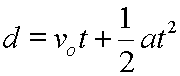## StatsMiniBlog: Significance tests. Step two.Now last time we examined the core question of ‘what is a statistical test asking’ with the answer  ‘what’s the likelihood that the results I’ve got from these two groups are different only because of the play of chance?’

Now depending on what sort of data you have will depend on what ‘the results from these two groups’ looks like. It might be that the data are continuous and Normal (so we need parametric tests), or continuous and non-Normal, or categorical (so we need non-parametric tests).

The choice of test, usually, falls out like this for continuous variables:

Repeated tests in same individuals               Tests in different individuals          Testing >2 groups

Parametric                                     paired t-test                                                  (unpaired) t-test                                 ANOVA

Non-Parametric                 Wilcoxon rank-sum test                               Mann-Whitney U test                      Kruskal-Wallis

With these tests you are essentially asking ‘how likely is it that the middleish value of the groups is different only because of the play of chance?’

For categorical variables you’ll be wanting the chi-squared test. (Note; χ2 is not just for squares – 2×2 tables – but can be 3×2, 4×6 or whatever.) What you’re asking here is “how likely is it that the proportion of items in each column, if we look at each row in turn, different only because of the play of chance?’

And, basically, that’s that.

– Archi

(Visited 105 times, 1 visits today)